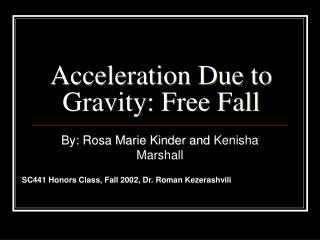# Acceleration Due to Gravity: Free Fall - PowerPoint PPT PresentationDownload PresentationAcceleration Due to Gravity: Free Fall

Acceleration Due to Gravity: Free FallDownload Presentation## Acceleration Due to Gravity: Free Fall

- - - - - - - - - - - - - - - - - - - - - - - - - - - E N D - - - - - - - - - - - - - - - - - - - - - - - - - - -
##### Presentation Transcript

1. Acceleration Due to Gravity: Free Fall By: Rosa Marie Kinder and Kenisha Marshall SC441 Honors Class, Fall 2002, Dr. Roman Kezerashvili

2. Objective • To verify that the displacement of a freely falling body released from rest is directly proportional to the square of the elapsed time. • To determine from the experiment the value for g, the acceleration due to gravity. • To verify that the time of free fall does not depend on the mass of the falling object.

3. Theory • Objects acted upon only by the force of gravity are in free fall. If we allow an object to fall in vacuum so that air resistance does not affect its motion, we find a remarkable fact, I all bodies, regardless of their size, shape or composition, fall at the same acceleration the same point near the Earth’s surface. • When an object is in free fall (whether up or down) objects are accelerated (or decelerated) at a constant rate by gravity, its magnitude, by the symbol g, and at or near the Earth’s surface g is approximately 9.81m/s^2. this is the standard for g.

4. Equations

5. Procedure • Part One • The free fall apparatus was previously set up. • The free fall pad was positioned under the release mechanism. • The steel ball was placed in the release mechanism and secured, but not yet released. • Connection to Digital Channel one as made on the Science Workshop Interface. • The distance of the free falling object was measured at 0.3m. • The ball was released and the data was recorded. • The ball was dropped at the same distance for three times, enabling us to calculate the mean. • These steps were carried out for several other heights: 0.5m, 0.7m, 0.9m, 1.1m, 1.3m, and 1.5m.

6. Part two • The same steps were repeated from part one except the height was changed to 1.0 meters and two balls of different weights were used in order to verify that the time of free falling objects do not depend on the mass of the falling objects.

7. Excuse me, No problem Professor Please, get to the point, we are running out of time

8. Data

9. Verification that free fall does not depend on the mass of the falling object.

10. Height vs. Time

11. Height vs. Time^2

12. GraphsVelocity vs. Time

13. Questions • A ball is dropped from the roof of a building. The ball strikes the ground after 6 s. How tall, in meters is the building?

14. More Questions • What is the magnitude of the ball’s velocity just before it reaches the ground?

15. Yes, More Questions. • A student throws a ball vertically downward from the top of the building. The ball leaves the thrower’s hand with a speed of 10m/s.What is the speed after falling for 1s, 2s, 3s? • How far does it fall in 1s, 2s, 3s? y1 = 10 + 4.9(9) y2 = 10 + 4.9(4) y3 = 10 + 4.9(9) y1 = 14.9m y2 = 29.6m y3 = 54.1m

16. Last Question! • What is the magnitude of its velocity after falling 10m?

17. Conclusion • The experiment was conducted successfully and we were able to obtain a value for g (the acceleration due to gravity) and compare it with the standard. This experiment has shown that the acceleration of free fall is independent of the mass of the falling object.

18. The End Thank You